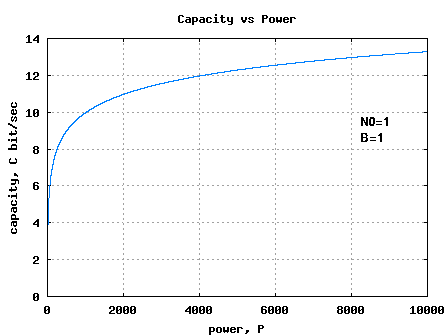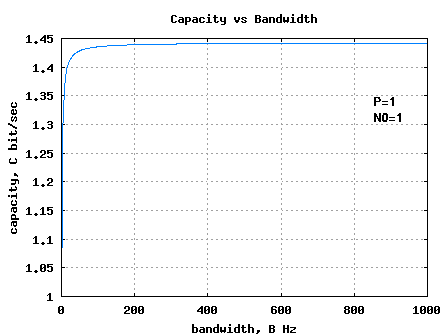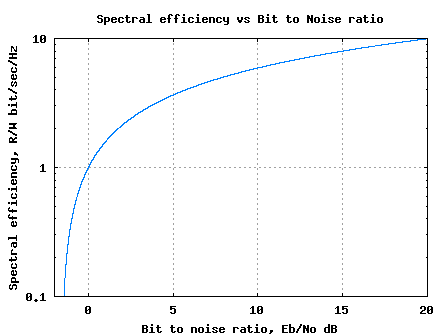(6 votes, average: 4.83 out of 5)Loading ...

Bounds on Communication based on Shannon’s capacity

by on June 18, 2008

This is the second post in the series aimed at developing a better understanding of Shannon’s capacity equation. In this post let us discuss the bounds on communication given the signal power and bandwidth constraint. Further, the following writeup is based on Section 12.6 from Fundamentals of Communication Systems by John G. Proakis, Masoud SalehiIn the first post in this series, we have discussed Shannon’s equation for capacity of band limited additive white Gaussian noise channel with an average transmit power constraint. The capacity is,

bits/second

where

is the capacity in bits per second, is the bandwidth in Hertz, is the signal power and is the noise spectral density.

Capacity with increasing signal power

Increasing the signal power will mean that we can split the signal level into more number of levels even while ensuring low probability of error. Hence increasing signal power will lead to more capacity. However, as the increase in capacity is a logarithmic function of power, the returns are diminishing.

Matlab/Octave script for plotting capacity vs power
B=1;
N0=1;
P= [0:10^4];
C = B.*log2(1+P./(N0*B));
plot(P,C); xlabel('power, P'); ylabel('bandwidth,B'); ylabel('capacity, C bit/sec'); title('Capacity vs Power')Figure: Capacity vs Power, keeping Noise and Bandwidth to unity

Can observe that increase in capacity is diminishing as we keep increase the value of power.

Capacity with increasing bandwidth

The second variable to play with is the bandwidth. Increasing the bandwidth has two effects:

1. More bandwidth means we can have more transmissions per second, hence higher the capacity.

2. However, more bandwidth also means that there is more noise power at the receiver.

The latter reduces the performance.

Let us try to evaluate the capacity equation when bandwidth tends to infinity i.e
.

From the Taylor series expansion, we know that

.

Applying this to the above equation,

.

This means that increasing bandwidth alone will not lead to increase of the capacity.

Matlab/Octave script for plotting capacity vs bandwidth
P = 1;
N0 = 1;
B = [1:10^3];
C = B.*log2(1+P./(N0*B));
plot(B,C)
xlabel('bandwidth, B Hz'); ylabel('capacity, C bit/sec'); title('Capacity vs Bandwidth')Figure: Capacity vs Bandwidth, keeping signal power and noise power to unity

Can observe that the maximum achievable capacity by increasing bandwidth is 1.44 times the value.

Capacity (in bit/sec/Hz) vs Bit to noise ratio (Eb/No)

From our discussion till now, we have understood that a practical communication should have a rate which is lower than capacity , i.e.

bits/second.

Dividing both sides of the equation by bandwidth ,

bits/second/Hz.

Further, from our discussion on Bit error rate for 16PSK modulation using Gray mapping, we know that symbol to noise ratio is times the bit to noise ratio, i.e.
.

Substituting this into the capacity equation,
bits/second/Hz.

For notational convenience, let us define as the spectral efficiency in bits/second/Hertz.

The above equation can be equivalently represented as,

.

In the above equation, when tends to zero, the bit to noise ratio should be,

.

(Thanks to L’Hospital’s rule).

This means that for reliable communication, we need to have or equivalently expressing in decibels, .

Matlab/Octave script for plotting the capacity in Bits/sec/Hz vs Bit to noise ratio
r = [0:.001:10];
Eb_No_lin = (2.^r -1)./r;
Eb_No_dB = 10*log10(Eb_No_lin);
semilogy(Eb_No_dB,r)
axis([-2 20 0.1 10]); grid on
xlabel('Bit to noise ratio, Eb/No dB'); ylabel('Spectral efficiency, R/W bit/sec/Hz')
title('Spectral efficiency vs Bit to Noise ratio')Figure: Spectral efficiency vs bit to noise ratio

The above plot captures the equation,

.

It divides the area into two regions:

(a) In the region below the curve, reliable communication is possible and

(b) in the region above the curve, reliable communication is not possible.

Closer the performance of a communication system is to the curve, more optimal is the system.

In the next post in this series, we will discuss the performance of various modulation schemes like BPSK, QPSK, QAM etc by mapping them into various points in the above plot.

Reference

[COMM-SYS-PROAKIS-SALEHI] Fundamentals of Communication Systems by John G. Proakis, Masoud SalehiD id you like this article? Make sure that you do not miss a new article by subscribing to RSS feed OR subscribing to e-mail newsletter. Note: Subscribing via e-mail entitles you to download the free e-Book on BER of BPSK/QPSK/16QAM/16PSK in AWGN.Sam April 28, 2011 at 10:30 am

Hi Krishna,

In the end of this tutorial you tell that “in the next post we will discuss the performance of various modulation schemes on the capacity vs bit error ratio curve”. Have you written a post on that? If not I am needling help to calculate the capacity of the AWGN channel for various modulation schemes like M-PSK and M-QAM. Can you tell me how to calculate that?Krishna Sankar May 26, 2011 at 5:34 amule August 18, 2010 at 5:11 pm

hi krishina this is very helpfull blog
thank youcommunications_engineer February 6, 2010 at 9:13 pm

Hello Krishna, how to plot the throughput against ber?

An un-verifiable source told me that

Is it Throughtput = (1 – BER)*Capacity?Krishna Sankar April 4, 2010 at 4:17 am

@communications_engineer: Am not sure. When talking about throughput (for eg. in wireless lan case etc), we need to account for preamble, media access overheads etc. But, in general the above equation seems to be rightAsad December 29, 2009 at 1:45 pm

Hi Krishna, I want to ask you whether there is a way to simulate channel capacity. Meaning if i wanted to actually see how much capacity the channel has based on the BER simulation of any system?donghak November 18, 2009 at 5:51 pm

sir.
I feel heavy ,not to sove 64QAM SER, BER……..
Please can you post me 64QAM matlab code?Krishna Sankar December 6, 2009 at 4:09 pm

I have discussed the M-QAM case, which you can adapt to 64-QAM.Arun kumar January 19, 2010 at 2:10 pm

sir,
Can you please tell me something about outage and ergodic capacity.I am doing project in physical layer network coding with diversity.SUBHA September 10, 2009 at 1:56 pm

Sir,

1.How to find the SNR of WiMAX?
2.Relation between capacity and PAPR of WiMAX?Krishna Sankar September 11, 2009 at 5:22 am

@SUBHA:
1/ SNR depends on the received signal power and the bandwidth of the receiver. For eg, if the received signal power is -80dBm and the received bandwidth is 20MHz (noise floor of -101dBm), then the SNR is 21dB
2/ No relation. Capacity corresponds to bits/seconds/Hz. PAPR is the peak to average power ratioJack October 22, 2010 at 8:51 am

Sir,why the SNR is related with the received bandwidth?carlos August 29, 2009 at 9:48 am

Hello. Can you explain me how to plot Nyquist channel capacity with MatLab or Octave?
I need some examples of if… if you can =)

ThanksKrishna Sankar September 7, 2009 at 5:19 am

@carlos: Nyquist capacity OR Shannon capacity?carlos September 11, 2009 at 3:49 pm

Nyquist =)basma May 13, 2009 at 4:55 am

i would like to ask if i want to draw a relation between R/W and Eb/No
acording to this equation : R/W = log2(1+ ((R/w)*(Eb/No))Krishna Sankar May 20, 2009 at 5:08 am

@basma: This plot is captured in the plot “Figure: Capacity vs Power, keeping Noise and Bandwidth to unity”. Agree?communicaitons engineer December 15, 2008 at 9:07 pm

Krishna start coding theory forum, it’d be a success

MY email is communications (underscore) engineer (at) yahoo (dot) comKrishna Sankar December 16, 2008 at 6:18 am